# TC Starter for waveform generator¶

In :
# Load Extensions

# cpu line profiler
# memory profiler
# Cython support

# Reload modules before executing user code

# setup backend for matplotlibs plots
%matplotlib inline

In :
# Imports
import pandas as pd
import numpy as np
import matplotlib.pyplot as plt
import seaborn as sns
from IPython.display import Audio
sns.set()

In :
def generateNoise(num_samples):
"""Generate random signal for each sample.

Using normal distribution instead of uniform distribution for random function

Args:
num_samples (int): number of samples

Returns:
ndarray: array of floats from standard normal distirbution
"""

return np.random.randn(num_samples)

In :
def generateSignal(time_samples,frequency,addNoise=True):
"""Generate sinusoidal data.

Generates pure sine waveform data and additional noise
can be added to the waveform.

Args:
time_samples (ndarray): time samples, for every point a signal data point is generated
freuency (int): signal frequency for one period

Returns:
ndarray: either pure siganl or pure signal with noise
"""

signal =  np.sin(2*np.pi*frequency*time_samples)

return signal + generateNoise(len(time_samples))
else:
return signal


In :
def generateTimeSamples(duration,rate):
"""Generate time series
The series is the len of duration*rate

Arags:
duration (int): signal duration is seconds
rate (int): sampling rate of the signal, how many points per second

Returns:
ndarray: Time series with a data point for each smapling point with in a second
"""
return np.arange(0,duration,1/rate, dtype=np.float64)

In :
def processTimeSeriesData(duration,rate,frequency,addNoise=True):
"""Process data"""

# generate time series
time_samples = generateTimeSamples(duration,rate)

# create pandas - init with time
df = pd.DataFrame(data=time_samples, columns=['time'])

# generate signal

# return data frame with signal
return df

In :
# set random seed for reproducibility
np.random.seed(1)

# length of sample in seconds
sample_duration = 2*60*60
# number of samples in a second
sample_rate = 44100
# sample oscillation with in a second
sample_frequency = 261.63 # Middle C since it sounds nice

In :
%lprun -f processTimeSeriesData mainDF_with_noise = processTimeSeriesData(sample_duration,sample_rate,sample_frequency,True)

Timer unit: 1e-06 s

Total time: 10.6309 s
File: <ipython-input-6-fe83befdfe2b>
Function: processTimeSeriesData at line 1

Line #      Hits         Time  Per Hit   % Time  Line Contents
==============================================================
2                                               """Process data"""
3
4                                               # generate time series
5         1    1050599.0 1050599.0      9.9      time_samples = generateTimeSamples(duration,rate)
6
7                                               # create pandas - init with time
8         1        579.0    579.0      0.0      df = pd.DataFrame(data=time_samples, columns=['time'])
9
10                                               # generate signal
11         1    9579749.0 9579749.0     90.1      df['signal'] = generateSignal(df['time'].values,frequency,addNoise)
12
13                                               # return data frame with signal
14         1          1.0      1.0      0.0      return df
In :
# filter and plot 1 second of data
ax = mainDF_with_noise[mainDF_with_noise.time <= 1].plot(kind='line',x='time',y='signal',ylim=(-6,6),grid=True)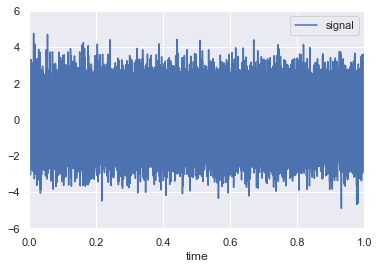In :
# filter and plot 0.1 second of data
ax =  mainDF_with_noise[mainDF_with_noise.time <= 0.1].plot(kind='line',x='time',y='signal',ylim=(-6,6),grid=True)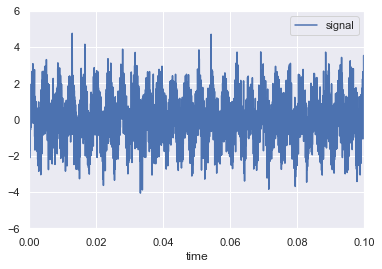In :
# Let's see how the signal looks in frequency domain
fqDF_noise = mainDF_with_noise[(mainDF_with_noise.time <= 5)] # look at only 5 seconds
fig, (ax1, ax2) = plt.subplots(nrows=2)
ax1.plot(fqDF_noise['time'], fqDF_noise['signal'])
ax2.specgram(fqDF_noise['signal'], NFFT=2048, Fs=sample_rate, noverlap=500)
plt.show()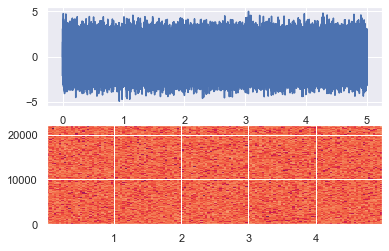In :
Audio(fqDF_noise['signal'],rate=sample_rate)

Out:
In :
%lprun -f processTimeSeriesData mainDF = processTimeSeriesData(sample_duration,sample_rate,sample_frequency,False)

Timer unit: 1e-06 s

Total time: 4.4634 s
File: <ipython-input-6-fe83befdfe2b>
Function: processTimeSeriesData at line 1

Line #      Hits         Time  Per Hit   % Time  Line Contents
==============================================================
2                                               """Process data"""
3
4                                               # generate time series
5         1    1140814.0 1140814.0     25.6      time_samples = generateTimeSamples(duration,rate)
6
7                                               # create pandas - init with time
8         1        503.0    503.0      0.0      df = pd.DataFrame(data=time_samples, columns=['time'])
9
10                                               # generate signal
11         1    3322085.0 3322085.0     74.4      df['signal'] = generateSignal(df['time'].values,frequency,addNoise)
12
13                                               # return data frame with signal
14         1          1.0      1.0      0.0      return df
In :
# filter and plot 1 second of data
ax = mainDF[mainDF.time <= 1].plot(kind='line',x='time',y='signal',ylim=(-6,6),grid=True)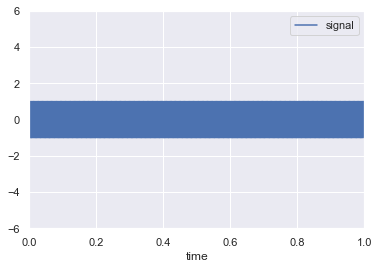In :
# filter and plot 0.1 second of data
ax =  mainDF[mainDF.time <= 0.1].plot(kind='line',x='time',y='signal',ylim=(-6,6),grid=True)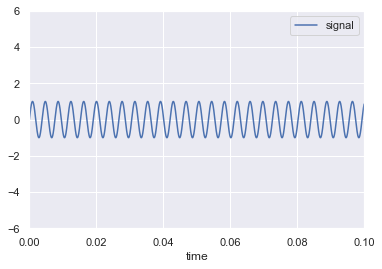In :
# Let's see how the signal looks in frequency domain
fqDF = mainDF[(mainDF.time <= 5)] # look at only 5 seconds
fig, (ax1, ax2) = plt.subplots(nrows=2)
ax1.plot(fqDF['time'], fqDF['signal'])
ax2.specgram(fqDF['signal'], NFFT=2048, Fs=sample_rate, noverlap=500)
plt.show()